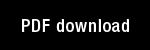Some networks that can learn, remember, and reproduce any number of complicated space-time patterns, I.

Author(s): Grossberg, S. |

Year: 1969

Citation: Journal of Mathematics and Mechanics, 19, 53-91

Abstract: 1. Introduction. This paper describes some networks 9R that can learn,simultaneously remember, and individually reproduce on demand any numberof spatiotemporal patterns (e.g., "motor sequences") of essentially arbitrary complexity. Because these networks are embedding fields, their behavior can be psychologically, neurophysiologically, and anatomically interpreted (,,, ). The network properties include the following.
(a) "Practice makes perfect".
(b) Memory of each pattern is essentially perfect if no competing experi-mental practice is imposed.
(c) New patterns can be learned without at all destroying the memory ofold patterns.
(d) All errors can be corrected.
(e) No "subject-induced" overt or covert practice is needed to ensure perfectmemory.
(f) Given a moderate amount of practice, memory spontaneously improves(i.e., "reminiscence" occurs).
(g) Memory is not destroyed by recall trials.
(h) Learning occurs by a mixture of respondant and operant conditioning,the operant effects including nonspecific arousal inputs in response to "novel"stimuli, and induced blocking of incoming inputs by inhibitory signals, leadingto "habituation" of repeated inputs. Both respondant and operant factors areunified into a single comprehensive learning mechanism.
(i) Only one "control neuron" is needed to activate reproduction of an entirespace-time pattern.
(j) The time needed to begin recall of a pattern can be made as small as weplease, and is independent of pattern complexity.
(k) The network is insensitive to wild "behaviorally irrelevant" oscillationsof inputs.
(l)The network dynamics, though nonlinear, can be analysed globally.
Networks that perform any number of complicated "reflex acts" (e.g., "walking", "clasping", "sniffing") will also be constructed, as a special case of thelearning networks. These "reflex" networks also satisfy (i)-(l) above.

Topics: Mathematical Foundations of Neural Networks, Models: Other,### Cross References

1. Embedding fields: A theory of learning with physiological implications
A learning theory in continuous time is derived herein from simple psychologicalpostulates. The theory has an anatomical and neurophysiological interpretation interms of nerve cell bodies, axons, synaptic knobs, membrane ... Article Details

2. A prediction theory for some nonlinear functional-differential equations, II: Learning of patterns
This paper studies the following system of nonlinear difference-differentialequations: [...](3)where i, j, k = 1, 2,..., n, and /3 0. We will establish global limit andoscillation theorems for the nonnegative solutions of ... Article Details

3. A prediction theory for some nonlinear functional-differential equations, I: Learning of lists
In this paper, we study some systems of nonlinear functional-differentialequations of the form
X(t) = AX(t) + B(X,) X(t - r) + C(t), t 0, (1)which ... Article Details

4. On the global limits and oscillations of a system of nonlinear differential equations describing a flow of a probabilistic network
1. INTRODUCTION: This paper considers various aspects of the global limiting and oscillatorybehavior of the following system of nonlinear differential equations.sx;(t) ... Article Details

5. On the variational systems of some nonlinear difference-differential equations
This paper studies the variational systems of two closely related systemsof nonlinear difference-differential equations which arise in prediction- andlearning-theoretical applications (, , [31). The first system is ... Article Details

Some networks that can learn, remember, and reproduce any number of complicated space-time patterns, II.

Author(s): Grossberg, S. |

Year: 1970

Citation: Studies in Applied Mathematics, 49, 135-166

Abstract: 1. Introduction - This paper describes some networks ..lf that can learn, simultaneously remember,and perform individually upon demand any number of spatiotemporal patterns(e.g., "motor sequences" and "internal perceptual representations") of essentiallyarbitrary complexity. Because these networks are embedding fields, they can begiven a suggestive psychological, neurophysiological, and anatomical interpre-tation (-).  describes some of the mathematical properties of thesenetworks using this heuristic interpretation. They include the following:
a) "Practice makes perfect".
b) Learning occurs by a mixture of operant and respondant conditioningfactors, which can include different network responses to "novel" vs."habituated" stimuli, the existence of "nonspecific arousal" and "internaldrive" stimuli, of "sensory" feedback due to prior "motor" outputs, andof "paying attention" by the network to those inputs which at any timehelp the network achieve its "goals".
c) New patterns can be learned without at all destroying the memory of oldpatterns.
d) All errors can be corrected.
e) Memory either decays at an exponential rate-which can be made arbitrarilysmall-or is perfect until "unrewarded" recall trials occur, during whichmemory is "extinguished". In both cases, "spontaneous recovery" andspontaneous improvement of memory (i.e., "reminiscence") can occur.
f) A single network "nerve", with sufficiently many "axon collaterals" activatedsuccessively by "avalanche conduction" can, in principle, learn an essentiallyarbitrarily complicated pattern, though in a rote way.
g) A concrete "stimulus sampling" operation occurs in the networks, andconcrete analogs of "stimulus sampling probabilities" exist.
h) The network is insensitive to wild "behaviorally irrelevant" oscillations ofinputs and often has a monotonic response to them.
i) Network dynamics can be globally analyzed.
 discusses a related class of networks whose memory is essentially perfecteven during recall trials.

Topics: Mathematical Foundations of Neural Networks, Models: Other,